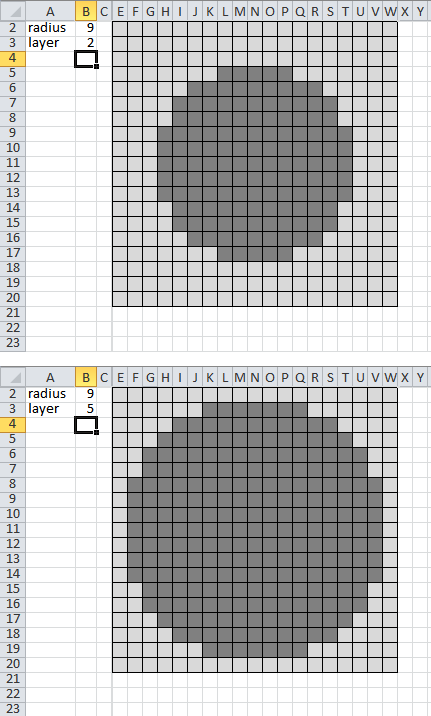All about flooble | fun stuff | Get a free chatterbox | Free JavaScript | Avatarsperplexus dot infoConstructing a Sphere (Posted on 2012-02-25)I would like to construct a sphere by gluing unit cubes together. I'm only attempting to approximate the shape of a sphere as closely as is possible with unit cubes.

Before I get out the glue, how would I go about creating a spreadsheet that would show me the quantity and placement of cubes in each cross-sectional layer? (think of an MRI scan cross-section)

For input, the spreadsheet should ask for the radius of the sphere to be built, as well as which layer of that sphere should be displayed.

There may be more than one way to accomplish this. Any spreadsheet that will allow me to build a sphere by displaying each layer of a sphere for a given radius is considered valid.

Here is an example of output:Hint: The formula for distance in 3 dimensions is:

`d=√[(x2-x1)2+(y2-y1)2+(z2-z1)2]`

Bonus question! Adjust your algorithm to display a hollow sphere (think of a ping pong ball, or a beach ball). Note: All cubes must be connected into one contiguous piece.

 No Solution Yet Submitted by Dustin Rating: 5.0000 (1 votes)Comments: ( Back to comment list | You must be logged in to post comments.)coming from behind| Comment 14 of 16 |Firstly this comment does rely on the reader having some concept of  spreadsheet 'mechanics'.  Secondly, this uses the idea of 'slicing' the object into odd numbers of layers which are from 0 to twice the layer value submiitted.

My solution, based upon interactions with Dustin, amounted to  a spreadsheet which looks rather like this:

Radius: "B1"      -20 -19 .........   0  ......... 19 20
Layer:   "B2" -20                                             Sum
-19                                             Sum
..                                              Sum
..                                              Sum
0                         X-Formula     Sum
..                                              Sum
..                                              Sum
19                                             Sum
20                                             Sum
Col Sum

X-Formula:
=IF((((((X\$1^2)+((\$B\$1-\$B\$2)^2))+(\$C22^2))^0.5)<(\$B\$1+0.5)),1," ")

Absolute cell recognition is essential for this to work (the '\$' symbol does that).

The formula is pasted into X22 which on this sheet has 'coordinates' X1 and C22 equal to zero .  That formula is then highlighted and "drag-copy" under the rules of the spreadsheet to fill the display grid; this does not take one operation. [X22 is (0,0) which has been noted].

The sum column tallies the "1" values in each row while the Col Sum tallies all of those within that column.

Edited on March 2, 2012, 6:50 am
 Posted by brianjn on 2012-03-02 06:06:33Please log in:

 Search: Search body:
Forums (0)